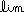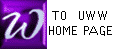AN EXAMPLE OF THE LIMIT METHOD
FOR FINDING THE DERIVATIVE OF f

Please review the general discussion before trying this example.

We pick a relatively simple example here : f(x) = x3 .

First recall the definition of "derivative" for any function f :

f ' (x) =h0
f(x+h) - f(x)
(x+h) - x

Now we interpret the functional notation for our particular function f(x) = x3 ;
at this point, you may want to review the binomial theorem :

f ' (x) =h0
 (x+h)3 - x3(x+h) - x

Now we must rewrite the fraction, since both top and bottom approach zero :

=h0
 [x3 + 3x2h + 3xh2 + h3] - x3(x+h) - x

Now we cancel two pairs of numbers, colored red above, and factor the top :

=h0
 3x2h + 3xh2 + h3h
=h0
 h[3x2 + 3xh + h2]h

At this point, both top and bottom are ZERO if h = 0. However, if h is NOT ZERO, we cancel the two red h above:

=h0
[3x2 + 3xh + h2]

And now the limit can be readily seen: the last two terms shrink to ZERO while the first term remains fixed.

= 3x2 + 0 + 0 = 3x2 .

Thus,   f ' (x) = 3x2